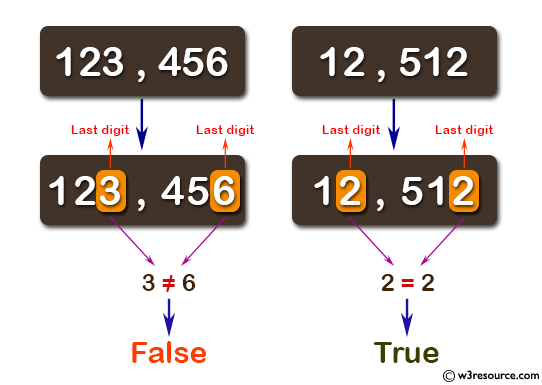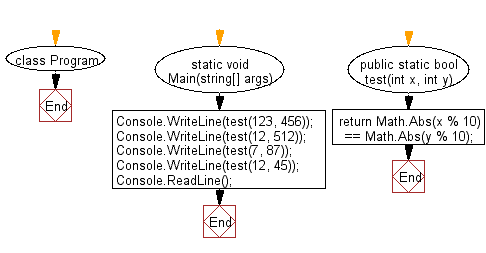﻿ C# - Check 2 non-negative integers have the same last digit# C# Sharp Basic Algorithm Exercises: Check whether two given non-negative integers have the same last digit

## C# Sharp Basic Algorithm: Exercise-23 with Solution

Write a C# Sharp program to check whether two given non-negative integers have the same last digit.

Pictorial Presentation:Sample Solution:-

C# Sharp Code:

``````using System;
namespace exercises
{
class Program
{
static void Main(string[] args)
{
Console.WriteLine(test(123, 456));
Console.WriteLine(test(12, 512));
Console.WriteLine(test(7, 87));
Console.WriteLine(test(12, 45));

}

public static bool test(int x, int y)
{
return Math.Abs(x % 10) == Math.Abs(y % 10);
}
}
}
```
```

Sample Output:

```False
True
True
False```

Flowchart:C# Sharp Code Editor:

Improve this sample solution and post your code through Disqus

What is the difficulty level of this exercise?

Test your Programming skills with w3resource's quiz.

﻿